Let’s say we have vectors `A1..An`. We know their dot products to `u` and `v`, but not `u` and `v` themselves. Can we estimate `u.v` (ie their cosine similarity)?

This could be handy in vector similarity systems. With just a few strategically selected reference points `A1..An` we might get an accurate view of `u.v` without storing the full vector. Or at least that’s the hope!

We first asked this question with just one reference point. Then we upgraded to two. Next we reach out with our feelings to get to all `An` reference points.

## Recap - using two reference points

With just one reference point, we can estimate `u.v` with just `u.A1 * v.A1`. Easy enough.

To add a second, `A2`, we figure out how much of `u.A2*v.A2` to include in `u.v`. We see how much of `A2` is already included in the original reference point `A1`. The leftover parts parts, we add to `u.v`.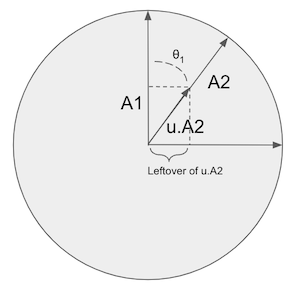Applying the trig knowledge you told your teacher you’d never need, you know that ‘leftover’ here is really ‘sin’. So we get:

``````u.v = u.A1*v.A1 + sin(θ1)*u.A2*v.A2
``````

Or put another way. if `A1` and `A2` are parallel, there’s no point in looking at the dot product `u.A2` - it’s just `u.A1` again. But as A2 rotates away, we add more and more of `u.A2` in.

## Up to 3 (or n) reference points

Going up to 3 (or n) dimensions, we need a similar procedure. However we need the leftovers of `A3` outside the plane created by `A1` and `A2`. That little slice of heaven shown below: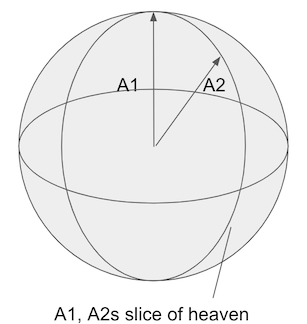The mathy way of defining the “plane” where `A1` and `A2` lie (really any `A1...An-1`) is called a span.

The problem becomes, how do we find an angle, `θ`, between `An` - not on the span - and its closest point on the span `A1...An-1`.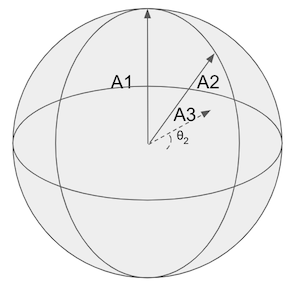If we find `θ` we can add another `+ sin(θ)*u.An*v.An` to the dot product and improve our estimate.

The ‘closest point’ of `An` on the span is known as a vector’s orthogonal projection, shown below: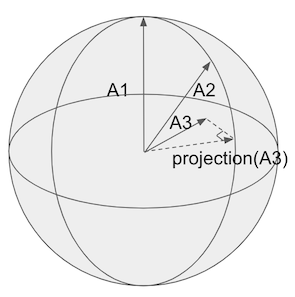The projection is just the other part of the triangle created by the ‘leftovers’ and An itself.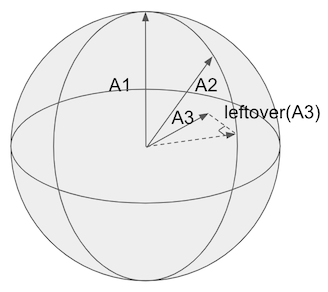Like thanksgiving, these leftovers are the juicy bits we want 🍗.

If we could find the projection, a little trig can get us `θ` - the angle between `An` and its `projection` - and thus `sin(θ)` - and viola leftovers 🍰!

How do we find the projection? Luckily there’s an existing algorithm. However, it requires an important input: the vectors describing the `A1..An-1` “slice of heaven” span must be made orthogonal to each other.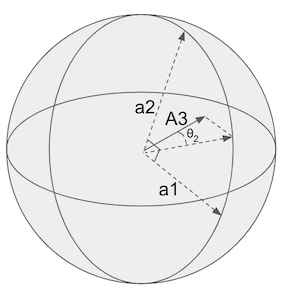To sum up, we need to follow two steps:

1. Go from our not orthogonal span `A1...An-1` to orthogonal vectors `a1...an-1` that also describe it.
2. Run the projection algorithm of An or each `a1..an-1`

There are many great articles describing each of these steps in isolation. We’ll not get into the weeds of each algorithm. But let’s walk through implementing each step in Python at a high level.

## To orthogonal vectors on span

Smart math people have gifted us algorithms for getting orthogonal vectors for a span. For example Gram Schmidt. There’s also a magic process in linear algebra called QR decomposition that does this for us (under the hood it runs an algorithm like Gram Schmidt for us).

QR decomposition turns a column matrix of our `A1..An-1`s into `Q` and `R`. We, care only about `Q` - it holds the vectors on the span orthogonal to each other. (Sorry `R` 😭.)

It’s a nice one liner for lazy programmers like me:

``````Q, R = np.linalg.qr(A)         # A here each column is A1...An-1
``````

Now each column of `Q` are orthogonal vectors still in `A1..An-1`’s slice of heaven:

Columns of Q:

a1 a2 an
a1 a2
a1 a2

## Getting the projection

The projection of unit vector `a` onto unit vector `b`:

``````def project_one(a, b):
"""Project a -> b ."""
return b * np.dot(a, b)
``````

(Be sure to see the definition for NON unit vectors, as we have to divide by b’s dot product with itself)

Then to get the full projection for all vectors in `a1...an` we subsequently add each projection:

``````    projection = []
for a in Q.T:
projection.append(project_one(v, a))
proj = sum(projection)
``````

With the vector `proj`, we can get the angle between vectors `proj` and `An` though their dot product. And add delicious leftovers🥪 to our `u.v` dot product estimate:

``````theta = math.acos(np.dot(proj, An))
u.v = A1.u*A1.v + ... + sin(theta)*An.u*An.v
``````

Nice, we can repeat this process for as many reference points as we have!

The next question, how accurate of a dot product can we get? And how many reference points would it require?

(The code here, with tests, can be found here )